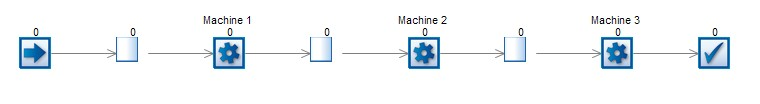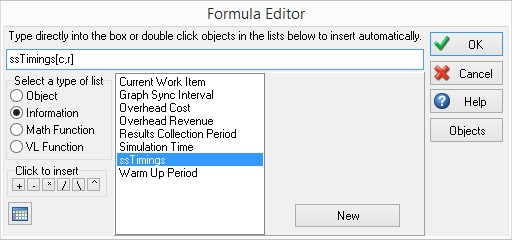## SIMUL8 Tip: Using the spreadsheet button within the formula editor

In this SIMUL8 tip, we will look at referencing a cell bayer levitra without a perscription in usa within an internal spreadsheet, using the spreadsheet button within the formula editor.

Let’s imagine that we have a factory, which is modeled using the simple simulation below:Within our simulation, we will have an internal spreadsheet which contains levitra cost low the timings for each of the machines. In this example, I will call this “ssTimings”. In most real-life examples, we would not simply have a spreadsheet with the machine name and the processing time:Instead, we are likely to have a complex spreadsheet, with a large amount of information stored about each machine:

If we want to use the ssTimings spreadsheet to set the processing time for each activity, we can use the button next to the “Average:” within the timing section of our ribbon, to open the formula editor.Within the formula editor, we can select the ssTimings object which populates the formula bar with “ssTimings[c,r]”. SIMUL8 needs us to specify the column number and row number within this formula, so that it knows which cell of the spreadsheet contains the timing information:If we have a simple spreadsheet, it can be easy to remember which cell contains the timing information, meaning that this information can be entered from memory; however, if our spreadsheet holds a lot of data and has multiple column headers, it can be tricky to remember the exact cell which contains the information we levitra mit rezept 10 mg preise require.

To assist us with this, if we place our cursor within the square brackets of the buy viagra in canada formula editor, the button in the lower left hand side of the dialog becomes active.Clicking generic levitra shipped from india on this button opens the ssTimings spreadsheet, allowing us to select the information which we require. For example, if we wanted to select the timing for Machine 1, by opening the spreadsheet, using this button, we can see that the information is viagra best buy contained within cell 4,2.We simply select the cell which we require and click “OK”. This populates the formula editor for us, with the selected cell reference: